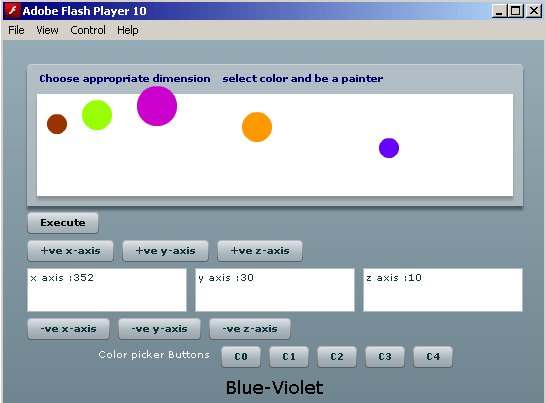Tutorials

# Flex Circle drawing example

Example below draws a circle on the panel background of your application. Circle and its properties like color, size and dimensions will completely depend on you as here you are both the artist and the button operator.

Example below draws a circle on the panel background of your application. Circle and its properties like color, size and dimensions will completely depend on you as here you are both the artist and the button operator.

# Flex circle drawing example

Example below draw circles on the panel background of your application. Circles drawn and its properties like color, size and dimensions will completely depend on you as here you are both the artist and the button operator. The circle is not a default feature, the instructions for drawing a circle is defined inside the Sprite class of flash.display package and so it has been imported in the application. The Sprite class elements demands UIComponent platform. In the program, we have used addChild function which is used by the UIComponents variable to call the sprite class variable inside them.

In the program code, addChild function is been used by two components, first by an UIComponent variable regularObject  to call  the flash.Sprite variable circle inside it and second by a flex container Panel whose id is bond077,  to call the UIComponent variable regularObject inside it.

In the program, a colorObject name variable is used which has value type Number.

Within the action script portion of file, two packages have been imported namely:  flash.display and mx.core. This import is done to get Sprite and UIComponent classes instances. In the code, a geometrical figure is created and for creating this figure a Sprite type variable has been created.  Now graphics feature of Sprite class provides two methods: beginFill() and drawCircle(). Among these methods the first one fills color in the figure and the second one creates the figure. Now the Sprite variable is called inside the UIComponent variable through addChild function and  the UIComponent variable is called inside the panel container through the panel id. Here calling UIComponent variable inside the panel container means figures will form on the panel background.

circle.mxml

 ```                  import flash.display.Sprite;   import mx.core.UIComponent;            public var x_axis:int = 0;     public var y_axis:int = 0;     public var z_axis:int = 0;               public function Xdimension():void{  x_axis = x_axis + 1;   }    public function Ydimension():void{   y_axis = y_axis + 1;     }    public function Zdimension():void{   z_axis = z_axis + 1;    }      public function minusXdimension():void{   x_axis = x_axis - 1;   }   public function minusYdimension():void{   y_axis = y_axis - 1;   }   public function minusZdimension():void{   z_axis = z_axis - 1;   }               public var objectColor:Number;                 public function C0():void{   objectColor = 0x6600FF;   }   public function C1():void{   objectColor = 0xFF9900;   }   public function C2():void{   objectColor = 0xCC00CC;   }   public function C3():void{   objectColor = 0x99FF00;   }   public function C4():void{   objectColor = 0x993300;   }                     public function createCircle():void{            var circle:Sprite;   circle = new Sprite();       circle.graphics.beginFill(objectColor);   circle.graphics.drawCircle(x_axis, y_axis, z_axis);            var regularObject:UIComponent;   regularObject = new UIComponent();   regularObject.addChild(circle);      bond077.addChild(regularObject);   }                        public function mouseOverEvent():void{   b0.addEventListener(MouseEvent.MOUSE_OVER, task_0);     b1.addEventListener(MouseEvent.MOUSE_OVER, task_1);     b2.addEventListener(MouseEvent.MOUSE_OVER, task_2);     b3.addEventListener(MouseEvent.MOUSE_OVER, task_3);     b4.addEventListener(MouseEvent.MOUSE_OVER, task_4);     }            public function task_0(e:Event):void{   lb.text = 'Blue-Violet';     }   public function task_1(e:Event):void{   lb.text = 'Intermediate (Red-Orange)';     }   public function task_2(e:Event):void{   lb.text = 'Secondary (Violet)';     }   public function task_3(e:Event):void{   lb.text = 'Intermediate (Yellow-Orange)';     }   public function task_4(e:Event):void{   lb.text = 'Intermediate (Red-Orange)';     }                        public function XDimensionDetails():void{   txt_0.text = 'x axis :' + x_axis.toString();     }    public function YDimensionDetails():void{   txt_1.text = 'y axis :' + y_axis.toString();     }    public function ZDimensionDetails():void{   txt_2.text = 'z axis :' + z_axis.toString();     }                                                                                                                                        .weisz {color : #000000}   Text {font-size : 18pt}           ```

circle.swf